# ANCOM-BC2 Tutorial

#### April 25, 2023

knitr::opts_chunk$set(message = FALSE, warning = FALSE, comment = NA, fig.width = 6.25, fig.height = 5) library(ANCOMBC) library(tidyverse) library(caret) library(DT) options(DT.options = list( initComplete = JS("function(settings, json) {", "$(this.api().table().header()).css({'background-color':
'#000', 'color': '#fff'});","}")))

# 1. Introduction

Analysis of Compositions of Microbiomes with Bias Correction 2 (ANCOM-BC2) is a methodology for performing differential abundance (DA) analysis of microbiome count data. This version extends and refines the previously published Analysis of Compositions of Microbiomes with Bias Correction (ANCOM-BC) methodology (Lin and Peddada 2020) in several ways as follows:

1. Bias correction: ANCOM-BC2 estimates and corrects both the sample-specific (sampling fraction) as well as taxon-specific (sequencing efficiency) biases.

2. Regularization of variance: Inspired by Significance Analysis of Microarrays (SAM) (Tusher, Tibshirani, and Chu 2001) methodology, a small positive constant is added to the denominator of ANCOM-BC2 test statistic corresponding to each taxon to avoid the significance due to extremely small standard errors, especially for rare taxa. By default, we used the 5-th percentile of the distribution of standard errors for each fixed effect as the regularization factor.

3. Sensitivity analysis for the pseudo-count addition: Like other differential abundance analysis methods, ANCOM-BC2 log transforms the observed counts. However, to deal with zero counts, a pseudo-count is added before the log transformation. Several studies have shown that differential abundance results could be sensitive to the choice of pseudo-count (Costea et al. 2014; Paulson, Bravo, and Pop 2014), resulting in an inflated false positive rate. To avoid such false positives, we conduct a sensitivity analysis and provide a sensitivity score for each taxon to determine if a particular taxon is sensitive to the choice of pseudo-count. The larger the score, the more likely the significant result is a false positive.

4. Multi-group comparisons and repeated measurements: The ANCOM-BC2 methodology extends ANCOM-BC for multiple groups and repeated measurements as follows:

• Multiple pairwise comparisons: When performning multiple pairwise comparisons, the mixed directional false discover rate (mdFDR) should be taken into account. The mdFDR is the combination of false discovery rate due to multiple testing, multiple pairwise comparisons, and directional tests within each pairwise comparison. For example, suppose we have five taxa and three experimental groups: g1, g2, and g3. Thus, we are performing five tests corresponding to five taxa. For each taxon, we are also conducting three pairwise comparisons (g1 vs. g2, g2 vs. g3, and g1 vs. g3). Within each pairwise comparison, we wish to determine if the abundance has increased or decreased or did not change (direction of the effect size). Errors could occur in each step. The overall false discovery rate is controlled by the mdFDR methodology we adopted from (Guo, Sarkar, and Peddada 2010; Grandhi, Guo, and Peddada 2016).

• Multiple pairwise comparisons against a pre-specified group (e.g., Dunnett’s type of test): We use the same set-up as in the multiple pairwise comparisons but use the Dunnett-type modification described in (Grandhi, Guo, and Peddada 2016) to control the mdFDR which is more powerful.

• Pattern analysis for ordered groups: In some instances, researchers are interested in discovering abundance patterns of each taxon over the ordered groups, for example, groups based on the health condition of subjects (e.g., lean, overweight, obese). In such cases, in addition to pairwise comparison, one may be interested in identifying taxa whose abundances are increasing or decreasing or have other patterns over the groups. We adopted methodologies from (Jelsema and Peddada 2016) to perform pattern analysis under the ANCOM-BC2 framework.

A clarification regarding Structural zeros: A taxon is considered to have structural zeros in some (>=1) groups if it is completely (or nearly completely) missing in these groups. For instance, suppose there are three groups: g1, g2, and g3. If the counts of taxon A in g1 are 0, but they are nonzero in g2 and g3, then taxon A will be considered to contain structural zeros in g1. In this example, taxon A is declared to be differentially abundant between g1 and g2, g1 and g3, and consequently, it is globally differentially abundant with respect to this group variable. Such taxa are not further analyzed using ANCOM-BC2, but the results are summarized in the overall summary.

# 2. Installation

if (!requireNamespace("BiocManager", quietly = TRUE))
install.packages("BiocManager")
BiocManager::install("ANCOMBC")

library(ANCOMBC)

# 3. Run ANCOM-BC2 on a simulated dataset

## 3.1 Generate simulated data

1. The simulated data contain: one continuous covariate: cont_cov, and one categorical covariate: cat_cov. The categorical covariate has three levels: “1”, “2”, and “3”. Let’s focus on the discussions on the group variable: cat_cov.

2. The true abundances are generated using the Poisson lognormal (PLN) model based on the mechanism described in the LDM paper (Hu and Satten 2020). The PLN model relates the abundance vector $$Y_i$$ with a Gaussian latent vector $$Z_i$$ for taxon $$i$$ as follows: $\text{latent layer: } Z_i \sim N(\mu_i, \Sigma_i) \\ \text{observation layer: } Y_i|Z_i \sim POI(N \exp(Z_i))$ where $$N$$ is the scaling factor. Because of the presence of a latent layer, the PLN model displays a larger variance than the Poisson model (over-dispersion). Also, the covariance (correlation) between abundances has the same sign as the covariance (correlation) between the corresponding latent variables. This property gives enormous flexibility in modeling the variance-covariance structure of microbial abundances since it is easy to specify different variance-covariance matrices in the multivariate Gaussian distribution.

3. Instead of specifying the variance-covariance matrix, we choose to estimate the variance-covariance matrix from a real dataset: the Quantitative Microbiome Project (QMP) data (Vandeputte et al. 2017). This dataset contains quantitative microbiome count data of 106 samples and 91 OTUs.

data(QMP)
set.seed(12345)
n = 150
d = ncol(QMP)
diff_prop = 0.1
lfc_cont = -1
lfc_cat2_vs_1 = -2
lfc_cat3_vs_1 = 1

# Generate the true abundances
abn_data = sim_plnm(abn_table = QMP, taxa_are_rows = FALSE, prv_cut = 0.05,
n = n, lib_mean = 1e8, disp = 0.5)
log_abn_data = log(abn_data + 1e-5)
rownames(log_abn_data) = paste0("T", seq_len(d))
colnames(log_abn_data) = paste0("S", seq_len(n))

# Generate the sample and feature meta data
# Sampling fractions are set to differ by batches
smd = data.frame(samp_frac = log(c(runif(n/3, min = 1e-4, max = 1e-3),
runif(n/3, min = 1e-3, max = 1e-2),
runif(n/3, min = 1e-2, max = 1e-1))),
cont_cov = rnorm(n),
cat_cov = as.factor(rep(seq_len(3), each = n/3)))
rownames(smd) = paste0("S", seq_len(n))

fmd = data.frame(seq_eff = log(runif(d, min = 0.1, max = 1)),
lfc_cont = sample(c(0, lfc_cont),
size = d,
replace = TRUE,
prob = c(1 - diff_prop, diff_prop)),
lfc_cat2_vs_1 = sample(c(0, lfc_cat2_vs_1),
size = d,
replace = TRUE,
prob = c(1 - diff_prop, diff_prop)),
lfc_cat3_vs_1 = sample(c(0, lfc_cat3_vs_1),
size = d,
replace = TRUE,
prob = c(1 - diff_prop, diff_prop))) %>%
mutate(lfc_cat3_vs_2 = lfc_cat3_vs_1 - lfc_cat2_vs_1)
rownames(fmd) = paste0("T", seq_len(d))

# Add effect sizes of covariates to the true abundances
dmy = caret::dummyVars(" ~ cat_cov", data = smd)
smd_dmy = data.frame(predict(dmy, newdata = smd))

log_abn_data = log_abn_data + outer(fmd$lfc_cont, smd$cont_cov)
log_abn_data = log_abn_data + outer(fmd$lfc_cat2_vs_1, smd_dmy$cat_cov.2)
log_abn_data = log_abn_data + outer(fmd$lfc_cat3_vs_1, smd_dmy$cat_cov.3)

# Add sample- and taxon-specific biases
log_otu_data = t(t(log_abn_data) + smd$samp_frac) log_otu_data = log_otu_data + fmd$seq_eff
otu_data = round(exp(log_otu_data))

# Create the tse object
assays = S4Vectors::SimpleList(counts = otu_data)
smd = S4Vectors::DataFrame(smd)
tse = TreeSummarizedExperiment::TreeSummarizedExperiment(assays = assays, colData = smd)

## 3.2 Run ancombc2 function

set.seed(123)
output = ancombc2(data = tse, assay_name = "counts", tax_level = NULL,
fix_formula = "cont_cov + cat_cov", rand_formula = NULL,
p_adj_method = "holm", pseudo = 0, pseudo_sens = TRUE,
prv_cut = 0.10, lib_cut = 1000, s0_perc = 0.05,
group = "cat_cov", struc_zero = FALSE, neg_lb = FALSE,
alpha = 0.05, n_cl = 2, verbose = TRUE,
global = FALSE, pairwise = FALSE,
dunnet = FALSE, trend = FALSE,
iter_control = list(tol = 1e-5, max_iter = 20,
verbose = FALSE),
em_control = list(tol = 1e-5, max_iter = 100),
lme_control = NULL, mdfdr_control = NULL,
trend_control = NULL)

res_prim = output$res tab_sens = output$pseudo_sens_tab

## 3.3 Sensitivity scores for “cat_cov”

1. Extreme values (>4) of the sensitivity score are observed.

2. Significant taxa with extreme sensitivity scores are likely false positives.

3. We empirically set the threshold for the sensitivity score as 5. Taxa with sensitivity scores larger than the specified threshold will be considered nonsignificant.

sens_cat = tab_sens %>%
transmute(taxon, sens_cat = cat_cov2) %>%
dplyr::left_join(res_prim %>%
transmute(taxon, diff_cat = diff_cat_cov2),
by = "taxon") %>%
mutate(group = "Cat2 vs. Cat1") %>%
bind_rows(
tab_sens %>%
transmute(taxon, sens_cat = cat_cov3) %>%
dplyr::left_join(res_prim %>%
transmute(taxon, diff_cat = diff_cat_cov3),
by = "taxon") %>%
mutate(group = "Cat3 vs. Cat1")
)
sens_cat$diff_cat = recode(sens_cat$diff_cat * 1,
1 = "Significant",
0 = "Nonsignificant")

fig_sens_cat = sens_cat %>%
ggplot(aes(x = taxon, y = sens_cat, color = diff_cat)) +
geom_point() +
scale_color_brewer(palette = "Dark2", name = NULL) +
facet_grid(rows = vars(group), scales = "free") +
labs(x = NULL, y = "Sensitivity Score") +
theme_bw() +
theme(axis.text.x = element_text(angle = 60, vjust = 0.5))
fig_sens_cat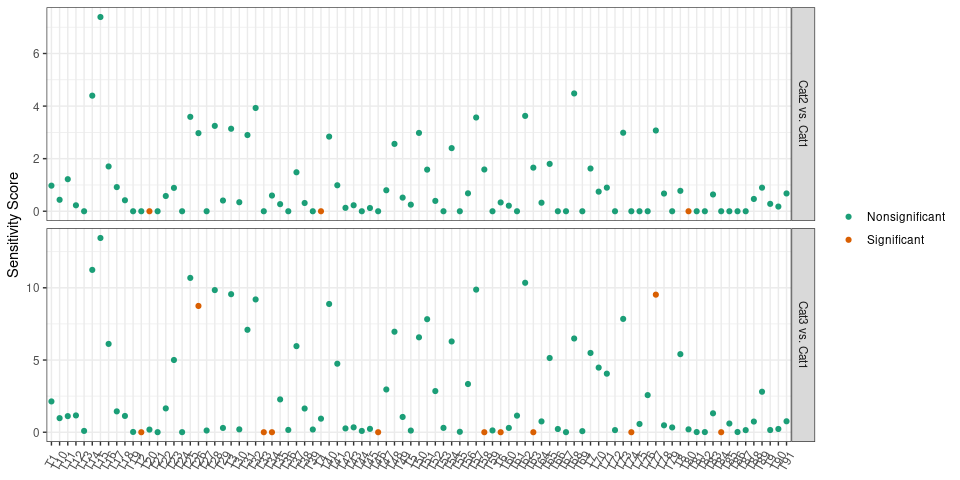sens_cut1 = 4
sens_cut2 = 4

## 3.4 Power and FDR

# Not considering the effect of pseudo-count addition
lfc = res_prim %>%
dplyr::select(starts_with("lfc"))
diff = res_prim %>%
dplyr::select(starts_with("diff"))
res_merge = (lfc * diff) %>%
mutate(taxon = res_prim$taxon) %>% dplyr::left_join(fmd %>% rownames_to_column("taxon"), by = "taxon") %>% transmute( taxon = taxon, est_cov2 = case_when( lfc_cat_cov2 > 0 ~ 1, lfc_cat_cov2 < 0 ~ -1, TRUE ~ 0), est_cov3 = case_when( lfc_cat_cov3 > 0 ~ 1, lfc_cat_cov3 < 0 ~ -1, TRUE ~ 0), true_cat2 = case_when( lfc_cat2_vs_1 > 0 ~ 1, lfc_cat2_vs_1 < 0 ~ -1, TRUE ~ 0), true_cat3 = case_when( lfc_cat3_vs_1 > 0 ~ 1, lfc_cat3_vs_1 < 0 ~ -1, TRUE ~ 0) ) # Cat 2 vs Cat 1 lfc_est = res_merge$est_cov2
lfc_true = res_merge$true_cat2 tp = sum(lfc_true != 0 & lfc_est != 0) fp = sum(lfc_true == 0 & lfc_est != 0) fn = sum(lfc_true != 0 & lfc_est == 0) power1_nosens = tp/(tp + fn) fdr1_nosens = fp/(tp + fp) # Cat 3 vs Cat 1 lfc_est = res_merge$est_cov3
lfc_true = res_merge$true_cat3 tp = sum(lfc_true != 0 & lfc_est != 0) fp = sum(lfc_true == 0 & lfc_est != 0) fn = sum(lfc_true != 0 & lfc_est == 0) power2_nosens = tp/(tp + fn) fdr2_nosens = fp/(tp + fp) # Considering the effect of pseudo-count addition res_merge2 = res_merge %>% dplyr::left_join(tab_sens, by = "taxon") # Cat 2 vs Cat 1 lfc_est = res_merge2$est_cov2 * (res_merge2$cat_cov2 < sens_cut1) lfc_true = res_merge2$true_cat2
tp = sum(lfc_true != 0 & lfc_est != 0)
fp = sum(lfc_true == 0 & lfc_est != 0)
fn = sum(lfc_true != 0 & lfc_est == 0)
power1_sens = tp/(tp + fn)
fdr1_sens = fp/(tp + fp)

# Cat 3 vs Cat 1
lfc_est = res_merge2$est_cov3 * (res_merge2$cat_cov3 < sens_cut2)
lfc_true = res_merge2$true_cat3 tp = sum(lfc_true != 0 & lfc_est != 0) fp = sum(lfc_true == 0 & lfc_est != 0) fn = sum(lfc_true != 0 & lfc_est == 0) power2_sens = tp/(tp + fn) fdr2_sens = fp/(tp + fp) tab_summ1 = data.frame(Comparison = c("Not considering the effect of pseudo-count addition", "Considering the effect of pseudo-count addition"), Power = round(c(power1_nosens, power1_sens), 2), FDR = round(c(fdr1_nosens, fdr1_sens), 2)) tab_summ2 = data.frame(Comparison = c("Not considering the effect of pseudo-count addition", "Considering the effect of pseudo-count addition"), Power = round(c(power2_nosens, power2_sens), 2), FDR = round(c(fdr2_nosens, fdr2_sens), 2)) tab_summ1 %>% datatable(caption = "Cat 2 vs Cat 1") tab_summ2 %>% datatable(caption = "Cat 3 vs Cat 1") # 4. Run ANCOM-BC2 on a real cross-sectional dataset ## 4.1 Import example data The HITChip Atlas dataset contains genus-level microbiota profiling with HITChip for 1006 western adults with no reported health complications, reported in (Lahti et al. 2014). The dataset is available via the microbiome R package (Lahti et al. 2017) in phyloseq (McMurdie and Holmes 2013) format. In this tutorial, we consider the following covariates: • Continuous covariates: “age” • Categorical covariates: “region”, “bmi” • The group variable of interest: “bmi” • Three groups: “lean”, “overweight”, “obese” • The reference group: “obese” data(atlas1006, package = "microbiome") tse = mia::makeTreeSummarizedExperimentFromPhyloseq(atlas1006) # subset to baseline tse = tse[, tse$time == 0]

# Re-code the bmi group
tse$bmi = recode(tse$bmi_group,
obese = "obese",
severeobese = "obese",
morbidobese = "obese")
# Subset to lean, overweight, and obese subjects
tse = tse[, tse$bmi %in% c("lean", "overweight", "obese")] # Note that by default, levels of a categorical variable in R are sorted # alphabetically. In this case, the reference level for bmi will be # lean. To manually change the reference level, for instance, setting obese # as the reference level, use: tse$bmi = factor(tse$bmi, levels = c("obese", "overweight", "lean")) # You can verify the change by checking: # levels(sample_data(tse)$bmi)

# Create the region variable
tse$region = recode(as.character(tse$nationality),
Scandinavia = "NE", UKIE = "NE", SouthEurope = "SE",
CentralEurope = "CE", EasternEurope = "EE",
.missing = "unknown")

# Discard "EE" as it contains only 1 subject
# Discard subjects with missing values of region
tse = tse[, ! tse$region %in% c("EE", "unknown")] print(tse) class: TreeSummarizedExperiment dim: 130 873 metadata(0): assays(1): counts rownames(130): Actinomycetaceae Aerococcus ... Xanthomonadaceae Yersinia et rel. rowData names(3): Phylum Family Genus colnames(873): Sample-1 Sample-2 ... Sample-1005 Sample-1006 colData names(12): age sex ... bmi region reducedDimNames(0): mainExpName: NULL altExpNames(0): rowLinks: NULL rowTree: NULL colLinks: NULL colTree: NULL ## 4.2 Run ancombc2 function set.seed(123) # It should be noted that we have set the number of bootstrap samples (B) equal # to 10 in the 'trend_control' function for computational expediency. # However, it is recommended that users utilize the default value of B, # which is 100, or larger values for optimal performance. output = ancombc2(data = tse, assay_name = "counts", tax_level = "Family", fix_formula = "age + region + bmi", rand_formula = NULL, p_adj_method = "holm", pseudo = 0, pseudo_sens = TRUE, prv_cut = 0.10, lib_cut = 1000, s0_perc = 0.05, group = "bmi", struc_zero = TRUE, neg_lb = TRUE, alpha = 0.05, n_cl = 2, verbose = TRUE, global = TRUE, pairwise = TRUE, dunnet = TRUE, trend = TRUE, iter_control = list(tol = 1e-2, max_iter = 20, verbose = TRUE), em_control = list(tol = 1e-5, max_iter = 100), lme_control = lme4::lmerControl(), mdfdr_control = list(fwer_ctrl_method = "holm", B = 100), trend_control = list(contrast = list(matrix(c(1, 0, -1, 1), nrow = 2, byrow = TRUE), matrix(c(-1, 0, 1, -1), nrow = 2, byrow = TRUE)), node = list(2, 2), solver = "ECOS", B = 10)) ## 4.3 Structural zeros tab_zero = output$zero_ind
tab_zero %>%
datatable(caption = "The detection of structural zeros")

tab_sens = output$pseudo_sens_tab tab_sens %>% datatable(caption = "Sensitivity Scores") %>% formatRound(colnames(tab_sens)[-1], digits = 2) ## 4.5 ANCOM-BC2 primary analysis Result from the ANCOM-BC2 methodology to determine taxa that are differentially abundant according to the covariate of interest. Results contain: 1) log fold changes, 2) standard errors, 3) test statistics, 4) p-values, 5) adjusted p-values, 6) indicators of whether the taxon is differentially abundant (TRUE) or not (FALSE). res_prim = output$res

### Results for age

#### Original results

df_age = res_prim %>%
dplyr::select(taxon, ends_with("age"))
df_fig_age = df_age %>%
filter(diff_age == 1) %>%
arrange(desc(lfc_age)) %>%
mutate(direct = ifelse(lfc_age > 0, "Positive LFC", "Negative LFC"))

### Results for BMI

df_bmi = res_prim %>%
dplyr::select(taxon, contains("bmi"))
df_fig_global = df_bmi %>%
dplyr::left_join(res_global %>%
transmute(taxon, diff_bmi = diff_abn)) %>%
dplyr::filter(diff_bmi == 1) %>%
mutate(lfc_lean = ifelse(diff_bmilean == 1,
lfc_bmilean, 0),
lfc_overweight = ifelse(diff_bmioverweight == 1,
lfc_bmioverweight, 0)) %>%
transmute(taxon,
Lean vs. Obese = round(lfc_lean, 2),
Overweight vs. Obese = round(lfc_overweight, 2)) %>%
pivot_longer(cols = Lean vs. Obese:Overweight vs. Obese,
names_to = "group", values_to = "value") %>%
arrange(taxon)

lo = floor(min(df_fig_global$value)) up = ceiling(max(df_fig_global$value))
mid = (lo + up)/2
fig_global = df_fig_global %>%
ggplot(aes(x = group, y = taxon, fill = value)) +
geom_tile(color = "black") +
scale_fill_gradient2(low = "blue", high = "red", mid = "white",
na.value = "white", midpoint = mid, limit = c(lo, up),
name = NULL) +
geom_text(aes(group, taxon, label = value), color = "black", size = 4) +
labs(x = NULL, y = NULL, title = "Log fold changes for globally significant taxa") +
theme_minimal() +
theme(plot.title = element_text(hjust = 0.5))
fig_global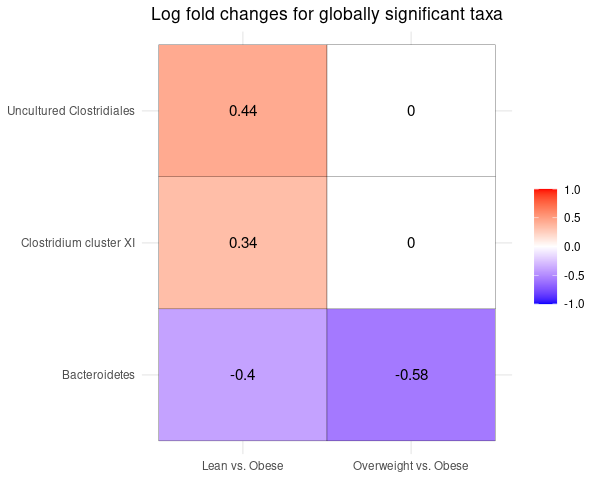### Pseudo-count sensitivity analysis for the global test

For the global test of bmi, no outlying sensitivity scores are observed. All significant taxa have low sensitivity scores.

sens_global = tab_sens %>%
transmute(taxon, sens_global = global) %>%
dplyr::left_join(res_global %>%
transmute(taxon, diff_global = diff_abn * 1),
by = "taxon")
sens_global$diff_global = recode(sens_global$diff_global,
1 = "Significant",
0 = "Nonsignificant")

fig_sens_global = sens_global %>%
ggplot(aes(x = taxon, y = sens_global, color = diff_global)) +
geom_point() +
scale_color_brewer(palette = "Dark2", name = NULL) +
labs(x = NULL, y = "Sensitivity Score") +
theme_bw() +
theme(axis.text.x = element_text(angle = 60, vjust = 0.5))
fig_sens_global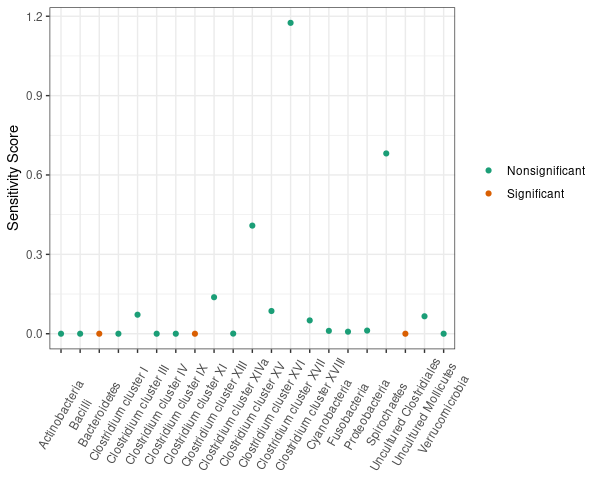## 4.7 ANCOM-BC2 multiple pairwise comparisons

ANCOM-BC2 multiple pairwise comparisons aim to determine taxa that are differentially abundant between any pair of two groups across three or more experimental groups, while controlling the mdFDR.

In this example, we want to identify taxa that are differentially abundant between any pair of two groups across “lean”, “overweight”, and “obese”. The result contains: 1) log fold changes, 2) standard errors, 3) test statistics, 4) p-values, 5) adjusted p-values, 6) indicators of whether the taxon is differentially abundant (TRUE) or not (FALSE).

### Results for BMI

df_fig_dunn = res_dunn %>%
dplyr::filter(diff_bmilean == 1 | diff_bmioverweight == 1) %>%
mutate(lfc_lean = ifelse(diff_bmilean == 1,
lfc_bmilean, 0),
lfc_overweight = ifelse(diff_bmioverweight == 1,
lfc_bmioverweight, 0)) %>%
transmute(taxon,
Lean vs. Obese = round(lfc_lean, 2),
Overweight vs. Obese = round(lfc_overweight, 2)) %>%
pivot_longer(cols = Lean vs. Obese:Overweight vs. Obese,
names_to = "group", values_to = "value") %>%
arrange(taxon)

lo = floor(min(df_fig_dunn$value)) up = ceiling(max(df_fig_dunn$value))
mid = (lo + up)/2
fig_dunn = df_fig_dunn %>%
ggplot(aes(x = group, y = taxon, fill = value)) +
geom_tile(color = "black") +
scale_fill_gradient2(low = "blue", high = "red", mid = "white",
na.value = "white", midpoint = mid, limit = c(lo, up),
name = NULL) +
geom_text(aes(group, taxon, label = value), color = "black", size = 4) +
labs(x = NULL, y = NULL, title = "Log fold changes as compared to obese subjects") +
theme_minimal() +
theme(plot.title = element_text(hjust = 0.5))
fig_dunn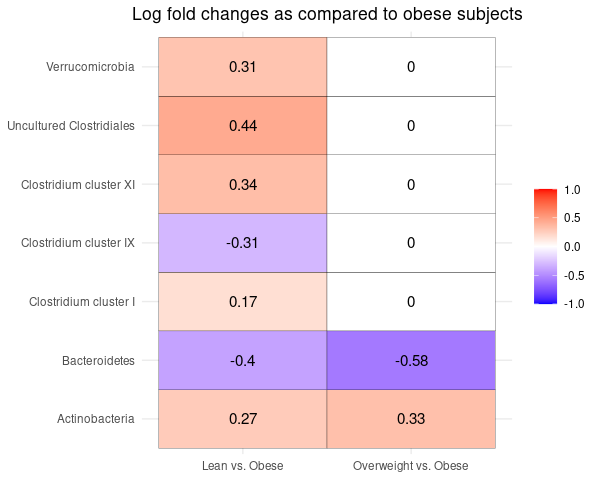### Pseudo-count sensitivity analysis for bmi

For the covariate of bmi, no outlying sensitivity scores are observed. All significant taxa have low sensitivity scores.

sens_dunn = tab_sens %>%
transmute(taxon, sens_bmi = bmilean) %>%
dplyr::left_join(res_dunn %>%
transmute(taxon, diff_bmi = diff_bmilean),
by = "taxon") %>%
mutate(group = "Lean vs. Obese") %>%
bind_rows(
tab_sens %>%
transmute(taxon, sens_bmi = bmioverweight) %>%
dplyr::left_join(res_dunn %>%
transmute(taxon, diff_bmi = diff_bmioverweight),
by = "taxon") %>%
mutate(group = "Overweight vs. Obese")
)
sens_dunn$diff_bmi = recode(sens_dunn$diff_bmi * 1,
1 = "Significant",
0 = "Nonsignificant")

fig_sens_dunn = sens_dunn %>%
ggplot(aes(x = taxon, y = sens_bmi, color = diff_bmi)) +
geom_point() +
scale_color_brewer(palette = "Dark2", name = NULL) +
facet_grid(rows = vars(group), scales = "free") +
labs(x = NULL, y = "Sensitivity Score") +
theme_bw() +
theme(axis.text.x = element_text(angle = 60, vjust = 0.5))
fig_sens_dunn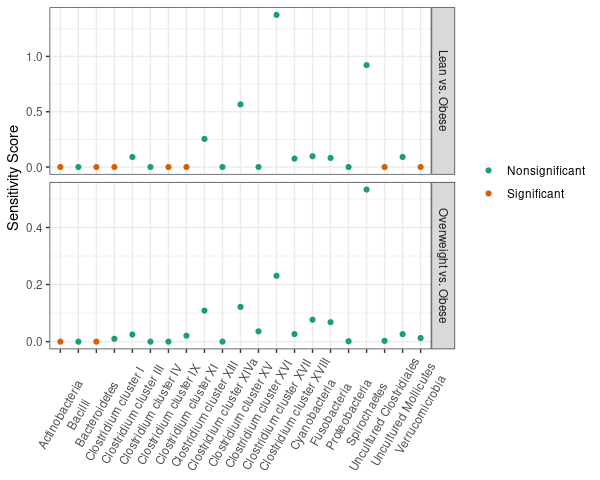## 4.9 ANCOM-BC2 pattern analysis

Sometimes the experimental groups are intrinsically ordered (e.g., in a dose-response study), and we would like to see if the microbial abundances show some patterns accordingly. Examples of patterns include: monotonically increasing, monotonically decreasing, and umbrella shape.

ANCOM-BC2 is able to identify potential patterns by testing the contrast: $Ax \ge 0$ where $$A$$ is the contrast matrix and $$x$$ is the vector of parameters.

For instance, in this example, we want to identify taxa that are monotonically increasing across “obese”, “overweight”, and “lean”. Note that “obese” is the reference group, and the parameters we can estimate are the differences as compared to the reference group, i.e., $x = (\text{overweight - obese}, \text{lean - obese})^T$ To test the monotonically increasing trend: $H_0: \text{obese} = \text{overweight} = \text{lean} \\ H_1: \text{obese} \le \text{overweight} \le \text{lean} \quad \text{with at least one strict inequality}$ We shall specify the contrast matrix $$A$$ as $A = \begin{bmatrix} 1 & 0 \\ -1 & 1 \end{bmatrix}$ Similarly, to test for the monotonically decreasing trend: $H_0: \text{obese} = \text{overweight} = \text{lean} \\ H_1: \text{obese} \ge \text{overweight} \ge \text{lean} \quad \text{with at least one strict inequality}$ We shall specify the contrast matrix $$A$$ as $A = \begin{bmatrix} -1 & 0 \\ 1 & -1 \end{bmatrix}$ To test for monotonic trend (increasing or decreasing), one should specify the node parameter in trend_control as the last position of x. In this example, the vector of parameters $$x$$ is of length 2, thus, the last position is 2. For testing umbrella shape, for instance, in this example: $H_0: \text{obese} = \text{overweight} = \text{lean} \\ H_1: \text{obese} \le \text{overweight} \ge \text{lean} \quad \text{with at least one strict inequality}$ one should set node as the position of the turning point of x. In this example, the turning position is overweight, thus, node = 1.

We will test both the monotonically increasing and decreasing trends in this example. The result contains: 1) log fold changes, 2) standard errors, 3) test statistics, 4) p-values, 5) adjusted p-values, 6) indicators of whether the taxon is differentially abundant (TRUE) or not (FALSE).

res_trend = output$res_trend ### Results for BMI Note that the LFC and SE for the reference group (“obese” in this example) will set to be 0s. df_fig_trend = res_trend %>% dplyr::filter(diff_abn) %>% transmute(taxon, lfc = lfc_bmioverweight, se = se_bmioverweight, q_val, group = "Overweight - Obese") %>% bind_rows( res_trend %>% dplyr::filter(diff_abn) %>% transmute(taxon, lfc = lfc_bmilean, se = se_bmilean, q_val, group = "Lean - Obese") ) df_fig_trend$group = factor(df_fig_trend\$group,
levels = c("Overweight - Obese", "Lean - Obese"))

fig_trend = df_fig_trend %>%
ggplot(aes(x = group, y = lfc, fill = group)) +
geom_bar(stat = "identity", position = position_dodge(), color = "black") +
geom_errorbar(aes(ymin = lfc - se, ymax = lfc + se), width = .2,
position = position_dodge(.9)) +
facet_wrap(vars(taxon), nrow = 2, scales = "free") +
labs(x = NULL, y = NULL, title = "Log fold change as compared to obese subjects") +
scale_fill_brewer(palette = "Set2", name = NULL) +
theme_bw() +
theme(plot.title = element_text(hjust = 0.5),
axis.text.x = element_blank(),
axis.ticks.x = element_blank(),
legend.position = c(0.92, 0.2))
fig_trend